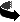Top: Forth IndexPrev: Storage, Strings & OutputNext: Afterword

Floating Point Numbers

Since integers can only be used on the default stack (not sure if this should be called the data stack or the control stack), there is a separate stack for floating point numbers and operations. The stack notation for the floating point stack is (F: -- ) (no space between the paren and the F:).

Floating point numbers may only be entered in the following format:

1234.5678e-3
The "e" is mandatory and floating point numbers will only be recognized if BASE is decimal. It also appears that f.s and f. will only display with an accuracy of three decimal places. Since it actually retains a more accurate value, there are special words for seeing these values, but they have not yet been implemented.

Floating Point Operations

Most of the commands you are used to can be prefixed by an "f" to obtain the floating point equivalent.

Integer Operation       Floating Point Operation
-----------------       ------------------------
+, -, *, /              f+, f-, f*, f/
variable, constant      fvariable, fconstant
., .s                   f., f.s, e.
!, @                    f!, f@
drop, swap, over        fdrop, fswap, fover
dup, rot, -rot          fdup, frot, f-rot
<, >, =, >=, <=         f<, f>, f=, f>=, f<=
0=, 0<>, 0<, 0>         f0=, f0<>, f0<, f0>
0<=, 0>=, <>            f0<=, f0>=, f<>
depth, clear            fdepth, fclear
There are many more, but that is enough for a comparison. Here is an example which uses the floating point stack exclusively. It is also a good example of a BEGIN-WHILE-REPEAT loop.

ok 3.1415926535E0  2E0  f* fconstant 2pi

ok : sine-table ( -- )  \ Print out the sine values between 0 and 2pi.
]  0E0                     ( angle )
]  begin
]      fdup 2pi  f<        ( angle end? )
]  while
]      fdup f.  fdup fsin  f.  cr
]      .1E0   f+           ( next-angle )
]  repeat  ;

ok sine-table
0.000 0.000
0.100 0.100
0.200 0.199
0.300 0.296
0.400 0.389
0.500 0.479
0.600 0.565
0.700 0.644
(...)
5.900 -0.374
6.000 -0.279
6.100 -0.182
6.200 -0.083
ok

Top: Forth IndexPrev: Storage, Strings & OutputNext: Afterword